Coincident current NOR gates
and combination of them to derive other logic gates

A practical guide of how to build your own.by SV3ORA
and Rory Mangles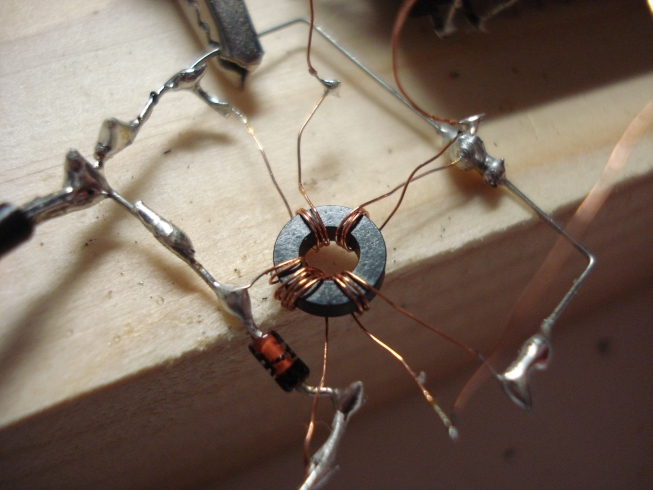This article presents my experiments in building a coincident current magnetic NOR gate and the rest of the derived gates. Unlike ordinary magnetic gates of the past, that used special toroidal or multi-aperture cores, the coincident current gate do not require the use of special transformers with square-loop BH curves. Practically, any core can be used to build the gate.

Nevertheless, using the coincident current technique, there are no "memory" effects and all the input signals must be applied to the gate simultaneously, for it to work correctly. There may be also fan-out problems by connecting more than one gates in series, unless you use some king of amplifier after the gate. Finally there may be some problems from the combination of more than one gates, as undesired currents may be induced to the windings of one gate from other gates. You have to keep these things in mind when building a multi-gate system.

Like NAND gates, NOR gates are so-called "universal gates" that can be combined to form any other kind of logic gate. For example, the first embedded system, Apollo Guidance Computer, was built exclusively from NOR gates, about 5,600 in total for the later versions. That is the reason why the NOR gate was chosen as a first demonstration for the experiment. Saying so, the NAND gate was found to be very difficult to design, using a single core. Next, let's talk about the NOR gate and how more of them can be combined, to form other gates. This information have been borrowed from wikipedia.

## NOR

A NOR gate is logically an inverted OR gate. By itself has the following truth table: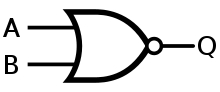Truth Table
Input A Input B   Output Q
0 0   1
0 1   0
1 0   0
1 1   0

A NOR gate is a universal gate, meaning that any other gate can be represented as a combination of NOR gates.

### NOT gate

This is made by joining the inputs of a NOR gate. As a NOR gate is equivalent to an OR gate leading to NOT gate, this automatically sees to the "OR" part of the NOR gate, eliminating it from consideration and leaving only the NOT part.

Desired Gate NOR Construction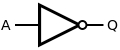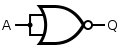Truth Table
Input A   Output Q
0   1
1   0

### OR

The OR gate is simply a NOR gate followed by another NOR gate.

Desired Gate NOR Construction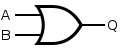Truth Table
Input A Input B   Output Q
0 0   0
0 1   1
1 0   1
1 1   1

### AND

An AND gate gives a 1 output when both inputs are 1; a NOR gate gives a 1 output only when both inputs are 0. Therefore, an AND gate is made by inverting the inputs to a NOR gate.

Desired Gate NOR Construction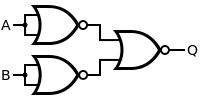Truth Table
Input A Input B   Output Q
0 0   0
0 1   0
1 0   0
1 1   1

### NAND

A NAND gate is made using an AND gate in series with a NOT gate:

Desired Gate NOR Construction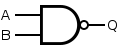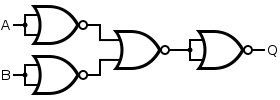Truth Table
Input A Input B   Output Q
0 0   1
0 1   1
1 0   1
1 1   0

### XOR

An XOR gate is made by connecting the output of 3 NOR gates (connected as an AND gate) and the output of a NOR gate to the respective inputs of a NOR gate. This expresses the logical formula (A AND B) NOR (A NOR B). This construction entails a propagation delay three times that of a single NOR gate.

Desired Gate NOR Construction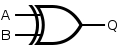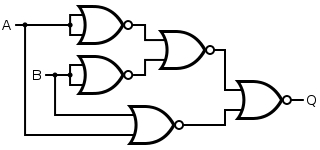Truth Table
Input A Input B   Output Q
0 0   0
0 1   1
1 0   1
1 1   0

### XNOR

An XNOR gate can be constructed from four NOR gates implementing the expression "(A NOR N) NOR (B NOR N) where N = A NOR B". This construction has a propagation delay three times that of a single NOR gate, and uses more gates.

Desired XNOR Gate NOR Construction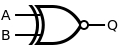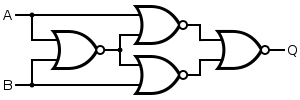Truth Table
Input A Input B   Output Q
0 0   1
0 1   0
1 0   0
1 1   1

Now that you have understood how other gates can be built using just NOR gates, let's try to build a single experimental coincident current magnetic NOR gate.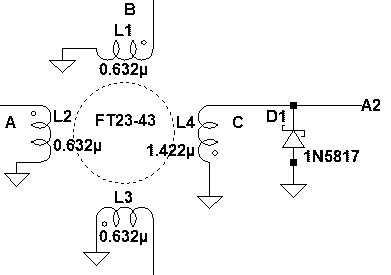This NOR gate uses a combination of a toroidal core, a special clock and a diode. The core used is the FT23-43, although any core can be adapted to the gate operation. Also any fast diode can be used at the output. The number of turns is experimental and it has to suit your needs. It also depends on your particular core, so a little bit of experimentation may be needed.

The coincident current gate operation is based on a simple principle. When two input windings carrying currents in the opposite directions pass through a toroidal core, their fields (induced into the core) cancel out, so no voltage is produced at the output winding. If the input windings carrying currents in the same direction (either positive or negative), their fields add up, and a voltage is produced at the output winding (positive or negative respectively).

Changing the currents direction, can be accomplished by changing the polarity of the voltage or by changing the winding phase. The second way was chosen, because it was easier for me to think logic one as a positive pulse instead of a negative one. The phase is indicated by the dots in the coils, in the gate schematic. Note that L1 and L2 have the same phase and L3 and L4 have also the same phase, but opposite than that of the L1 and L2.

To understand the operation of the gate, it's truth table must be presented. Remember, transformers are AC devices, meaning that they can operate either on continuous AC or pulse mode, not DC. This gate operates on pulses, thus logic one means a positive pulse and logic zero, no pulse at all. The truth table of the gate is shown below.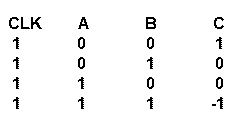A, B are opposite phase (negative polarity) to CLK

The theoretical challenge with such a gate, is to achieve only two absolute output states by adding or subtracting input voltages that exhibit only two states (e.g. 0v and 1v). To explain that better, let's ignore the CLK for a while and assume that A and B are of the same phase. Adding them, outputs 0, 1 and 2 (three absolute states). Subtracting them, outputs 0, -1 and -2. Now let's assume that A and B are of opposite phase. Subtracting them, outputs 0, 1 and 2. Adding them, outputs 0, -1, and 1. Apart from the latter case, there are always three output states generated. The latter case has two absolute states, but it fails to meet the specifications of a NOR gate.

The solution to the problem is another winding (L3), which acts as a clock pulse. This winding current combines with the input windings currents so that it generates only two absolute output states, 0 and 1/-1. The -1 pulse in the truth table should be 0, for correct operation of the gate. To be able to eliminate this negative occurring pulse out of the gate, a single diode can be used, that lets only the positive pulses out and cuts-off any negative pulses (convert a -1 state to 0). This simple trick, allows very easily to transform two absolute states (0, 1 and -1) to two real states (0 and 1). This was also the main reason why it was decided to use voltage level 0V, to represent logic zero and voltage level +V (any positive voltage), to represent logic one.

Considering the schematic and the truth table, windings A and B are of different phase than that of windings CLK and C. Thus, you may think of them as negative numbers, 0 and -1, where a 0 and a 1 respectively occurs. Now let's do the calculations for the CLK, A, B and C accordingly. 1+0+0=1, 1+0+(-1)=0, 1+(-1)+0=0, 1+(-1)+(-1)=-1. The latter negative pulse is blocked by the diode and it is read as 0 at the output. A successful NOR gate has been created.

As said before, transformers are AC devices, meaning that they can operate either on continuous AC or pulse mode, not DC. This means that if the CLK signal is a square wave, the transformer will respond to both the rising edge of the pulse (from 0v to +v) and the falling edge (from +v to 0v). In the falling edge, it would output a negative pulse, which would interfere with the inputs pulses in an undesirable way. Since a indefinitely continuous positive ramp signal is impossible, the only way to cope with this problem, is to use a reverse sawtooth waveform for the CLK signal.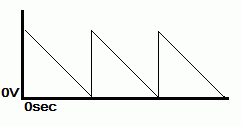At the beginning of the clock waveform, there is a rapid rising edge, which induces a high positive current to the CLK. The falling edge of the clock waveform is much more smoother and this induces a negative current to the CLK, but much more attenuated. The negative current cannot be entirely eliminated but it stays at low levels, so as not to interfere with the gate operation.

Note that the clock and the inputs are assumed to be applied simultaneously to the gate. In conjunction to logic based on square loop cores, there is no storage mechanism here and everything occurs in one clock cycle. Also, the clock and the input signals are assumed to be ideally of the same waveform shape, for the gate to operate correctly.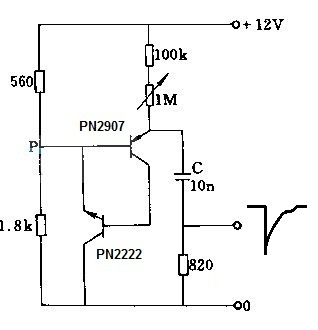A very simple reverse sawtooth generator can be built by just two complementary transistors. The circuit above, generates reverse sawtooth negative pulses for testing purposes. The output waveform is not linear and it is more like a capacitor discharge waveform, but it is ok for our testing purposes. Negative pulses is not of a problem. It just requires the windings on the transformer to be connected in reverse polarity. A much better better positive linear ramp circuit is described in this article.A prototype of the NOR gate was built in air style, without any pcb of fancy stuff. Picture above, shows the windings phase and where the signals should be fed. Remember, we are using a negative reverse sawtooth pulse for all the signals. The perimeter wire is the GND. All the signals to the gate (CLK, input A, input B) are fed using 10nF capacitors. All these capacitors are connected at their other end to the output of the same generator, therefore all pulses occur simultaneously. To achieve the same output level as the input levels, A is 3 turns, B is 3 turns, CLK is 3 turns and the output winding is 7 turns. At this point, I used a 1N914 diode for testing purposes.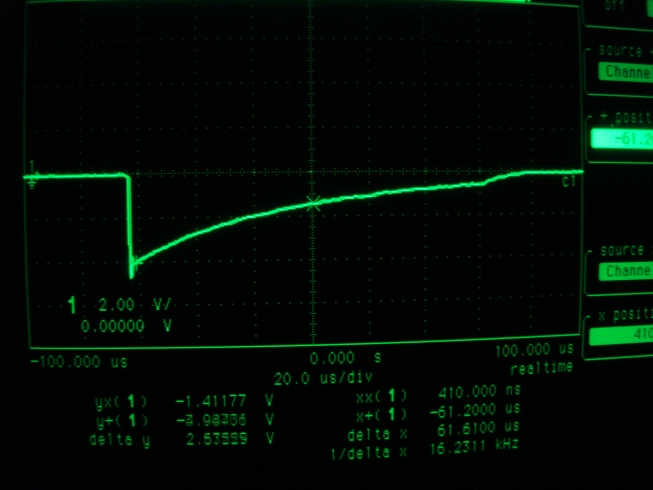The generator is connected to the scope above, showing a rapid increase of the negative pulse (5v), followed by a smooth and long time decay.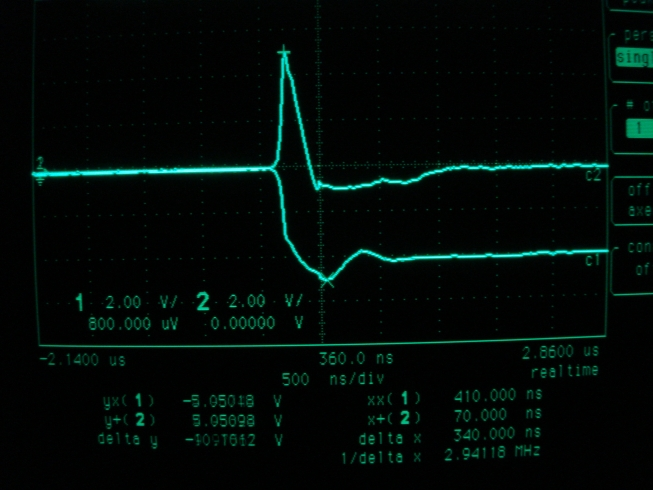Testing the gate above, shows the result when the inputs are both 0. The bottom trace is the CLK and the top trace, the gate output. Only the clock produces an output now.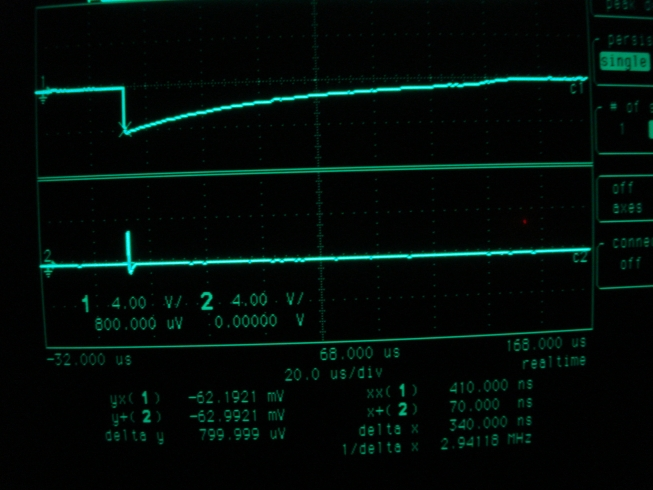Picture above, shows the comparison between the CLK pulse (top trace) and the output pulse (bottom trace) when the inputs are both 0. The transformer responds with a very sharp pulse compared to the CLK.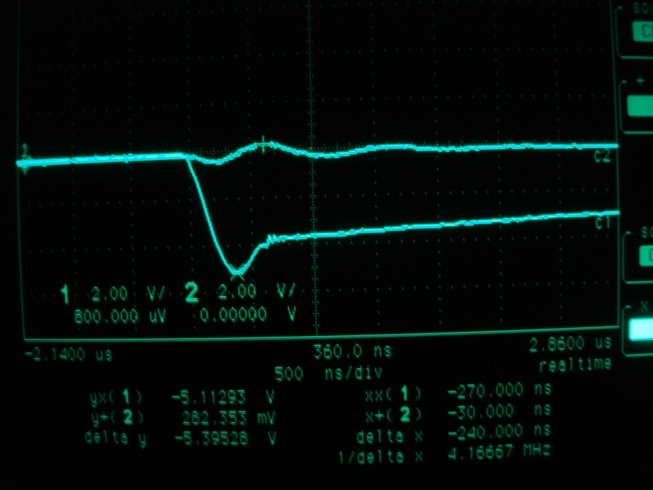Picture above, shows the result when the inputs are A=1 and B=0. The bottom trace is the CLK and the top trace, the gate output.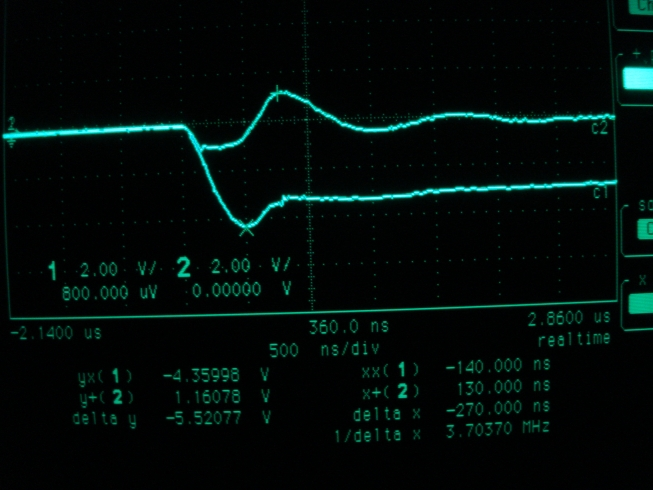Picture above, shows the result when the inputs are both 1. The bottom trace is the CLK and the top trace, the gate output.

To be continued...

Back to main site